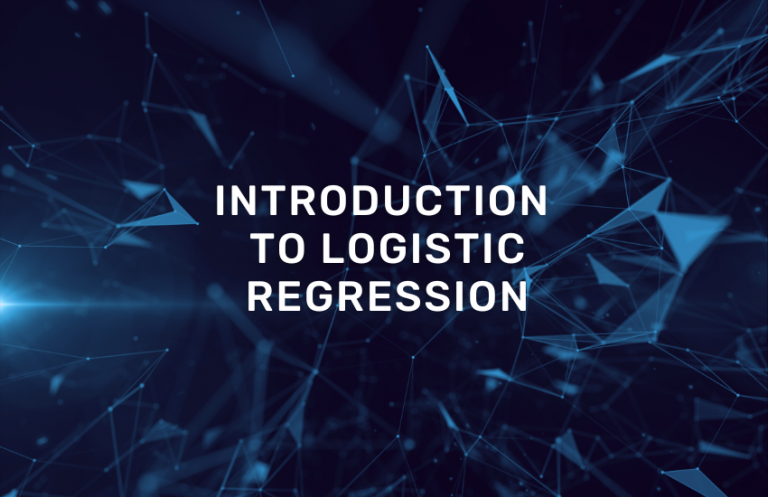# Carlos Rodriguez

## Introduction to Logistic RegressionIntroduction Logistic regression is a statistical methodology that allows modeling the relationships between a binary categorical variable and a set of explanatory variables. Specifically, it models the probability that an observation belongs to one of the categories of that binary…